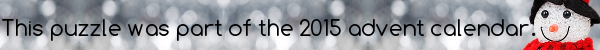mscroggs.co.uk
mscroggs.co.uksubscribe

# Puzzles

## 10 DecemberThis number is divisible by 2. One more than this number is divisible by 3. Two more than this number is divisible by 5. Three more than this number is divisible by 7. Four more than this number is divisible by 11. Five more than this number is divisible by 13.

## Archive

Show me a random puzzle
▼ show ▼